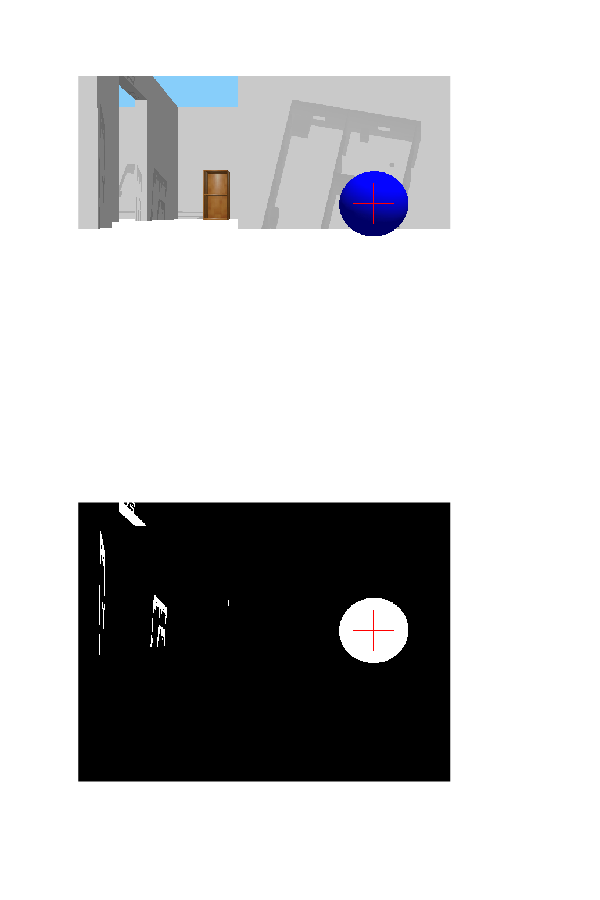# Track and Follow an Object Using a TurtleBot

This example shows how to track an object based on color using a TurtleBot® robot connected via a ROS network. ROS Toolbox Support Package for TurtleBot-Based Robots enables you to capture images to find an object in the environment and send velocity commands to navigate toward the object. To follow the object, you use the `getColorImage` and `setVelocity` functions along with image processing techniques.

### Connect to Robot

Connect to your TurtleBot robot using its specific IP address. Your simulated or real TurtleBot must be on the same ROS network as the computer running MATLAB®.

```ipaddress = '192.168.192.130'; % IP address of your robot tbot = turtlebot(ipaddress,11311); tbot.Velocity.TopicName = '/cmd_vel';```

### Track Object

To detect the example object, a blue ball, you must specify some of its properties. You use these properties to process the images you capture. This example uses Image Processing Toolbox™ to run color and blob detection algorithms on each image to find the ball.

Specify the properties of the ball shown, substituting in values that are tuned for your environment. The values given are optimal for the Gazebo simulation detailed in Get Started with Gazebo and Simulated TurtleBot.

```blueBallParams.blueMax = 120; % Maximum permissible deviation from pure blue blueBallParams.darkMin = 30; % Minimum acceptable darkness value```

Get an image off the TurtleBot.

`latestImg = getColorImage(tbot);`

Use these two example helper functions to get and plot the location of the ball in the image. These functions use `regionprops` from Image Processing Toolbox to get the location and size of the ball. The first image is the output from the TurtleBot with the object location overlaid on it. The second image is the binary image, `ballImage`, for detecting blue in the image based on `blueBallParams`. If the ball does not show up as white with limited background in this binary image, try tuning the `blueBallParams` values.

```[position,~,ballImage] = exampleHelperTurtleBotFindBlueBall(latestImg,blueBallParams,false); exampleHelperTurtleBotPlotObject(latestImg,ballImage,position);```### Follow Object Using Velocity Commands

After you tune the tracking of the object in the image, you can set up a basic control algorithm for sending commands to the TurtleBot. This example uses basic logic with singular linear and angular velocity commands to get the robot to follow the ball. For a more advanced control scheme, see Track and Follow an Object.

Get a new image and store the image size. Recalculate the position and size of the object.

```latestImg = getColorImage(tbot); [height,width] = size(latestImg); [position,~] = exampleHelperTurtleBotFindBlueBall(latestImg,blueBallParams,false);```

Generate angular and linear velocities based on the position and size of the ball. If no object is found, spin and search for the ball (positive angular velocity only).

• If the ball is on the far left side of the screen, the robot needs to turn left (positive angular velocity). The same is true for the right side (negative angular velocity).

• If the ball is too small in the image, the robot must move closer (positive linear velocity). If the ball is too large, the robot must move farther away (negative linear velocity).

Finally, send the linear and angular velocities using `setVelocity` and `pause` to wait for the command to send. Iterate for 100 cycles to test tracking.

```% Parameters for ball position and size horizontalTolerance = 20; sizeGoal = 70; sizeTolerance = 5; for i = 1:100 % Get latest image, ball postion, and ball size. latestImg = getColorImage(tbot); [height,width] = size(latestImg); [position,ballSize] = exampleHelperTurtleBotFindBlueBall(latestImg,blueBallParams,false); % Initialize velocities to zero. linearVel = 0; angularVel = 0; % Left and right controls if isempty(position) angularVel = 0.5; linearVel = 0; elseif (position(1) > (width/2)-horizontalTolerance) angularVel = 0.2; elseif (position(1) < (width/2)+horizontalTolerance) angularVel = -0.2; end % Forward and back control if isempty(ballSize) angularVel = 0.5; linearVel = 0; elseif ballSize > sizeGoal + sizeTolerance linearVel = - 0.1; elseif ballSize < sizeGoal - sizeTolerance linearVel = 0.1; end % Send velocity commands and wait for commands to send. setVelocity(tbot,linearVel,angularVel) pause(0.2) end```

To verify the algorithm is successful, capture an image and use the help functions to track and plot the position once more. The TurtleBot focuses on the ball, assuming it found the ball during its search. Based on the Gazebo simulated world in this example, a final image capture shows the ball right in front of the robot.

```latestImg = getColorImage(tbot); [c,ballSize,ballImage] = exampleHelperTurtleBotFindBlueBall(latestImg,blueBallParams,false); exampleHelperTurtleBotPlotObject(latestImg,ballImage,c); ```### Improvement Suggestions

This example uses simple sensor feedback and control parameters for tracking and following an object. It works well in a simulated environment due to its low level of risk to hardware and real environments. However, it does not factor in the bump or clip sensors, which can help with collisions or terrain changes. Dynamically adjusting the velocity commands and factoring in previous states can improve the movements of the TurtleBot.

To improve this example for real-world applications, consider using more advanced methods of sensing and control for real robots that have more robust handling of sensor data. See Track and Follow an Object for a more advanced example that uses a PID controller and other robot sensors.